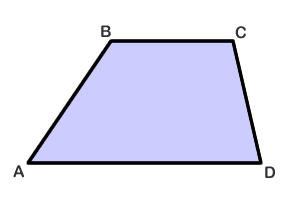Trapezoid
Parallelogram
Rhombus
Rectangle
Squares
100

What is a Trapezoid?

A shape with at least 1 pair of parallel sides

100

What is a parallelogram?

a shape where opposite sides are equal, and parallel, and opposite angles are equal

100

What are the propertys that a rhombus has?

diagnols perpendicular, diagnols bisect, all sides equal, everything a parallelogram has

100

propertys of a rectangle

all angles are right, diagnols are equal, and everything a parallelogram has

100

What are 2 things a square must have?

all right angles and all equal sides

200

Is a trapezoid a parallelogram?

No, a trapezoid is not a parallelogram but a parallelogram is a trapezoid

200

Quadrilateral abcd has a transversal, from ab to cd and ca is parallel to db. Opposite sides are equal, is this a parallelogram?

yes

200

What makes the diagonal of a rhombus special

They are perpendicular and Angle bisectors

200

Why is a rectangle not a square

Because square has all sides equal

200

is a square a trapazoid parallellogram, rectangle, and rhombus?

yes a square is the all mighty shape

300

Given angle b is 108 and abcd is a trapazoid, what is the measure of angle a?angle a = 180 - 108 = 72

300

is a hexagon a parallelogram?

No a parallelogram must be a quadrilateral

300

Is a rectangle a rhombus

No it does not have all equal sides

300

Why is it that when you have one right angle in a parallelogram it’s automatically a rectangle

Because angle pairs are supplementary

300

what makes the diagnols of a square special

they bisect, they are equal, they are perpendicular, and they are angle bisectors

400

A quadrilateral with 2 equal sides and 1 pair of opposite interior angles, what shape is this?

a trapezoid

400

How would you prove a parallelogram is a parallelogram in cord geometry?

Opposite sides have same slope

400

Parallelogram abcd has all equal sides, and all equal angles. Is this a rhombus?

No it’s a square because rhombus don’t have all equal angles

400

Parallelogram abcd has diagonals that bisect and are equal this shape also has one right angle. What shape is it?

Rectangle

400

what shape is this? a rhombus with equal diagonals and right angles

a square

500

Quadrilateral RSTU has vertices at R(-4,4), S(2,7),T(5,2), and U(-7,-4). What shape is this and do any of the slopes form a perpendicular line?

500

diffrence between a trapezoid and a parallelogram

trapazoid has one pair of parallel sides

500

How would you prove a parallelogram is a rhombus using cord geometry?

Opposite sides will have equal slope and using distance formula all sides should be the same distance.

500

How would you prove a rectangle In cord geometry?

Parallel sides and non parallel sides are perpendicular

500

how would you prove a square in cord geometry?

all opposing sides have equal slope and all sides have the same distance and non opposing sides are perpendicular

Click to zoom